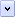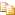Recursion is an important programming technique. It is used to have a function call itself from within itself. One example is the calculation of factorials. The factorial of 0 is defined specifically to be 1. The factorials of larger numbers are calculated by multiplying 1 * 2 * ..., incrementing by 1 until you reach the number for which you are calculating the factorial.

# An Example of Recursion

The following paragraph is a function, defined in words, that calculates a factorial.

"If the number is less than zero, reject it. If it is not an integer, round it down to the next integer. If the number is zero, its factorial is one. If the number is larger than zero, multiply it by the factorial of the next lesser number."

To calculate the factorial of any number that is larger than zero, you need to calculate the factorial of at least one other number. The function you use to do that is the function you're in the middle of already; the function must call itself for the next smaller number, before it can execute on the current number. This is an example of recursion.

Recursion and iteration (looping) are strongly related - anything that can be done with recursion can be done with iteration, and vice-versa. Usually a particular computation will lend itself to one technique or the other, and you simply need to choose the most natural approach, or the one you feel most comfortable with.

Clearly, there is a way to get in trouble here. You can easily create a recursive function that does not ever get to a definite result, and cannot reach an endpoint. Such a recursion causes the computer to execute a so-called "infinite" loop. Here's an example: omit the first rule (the one about negative numbers) from the verbal description of calculating a factorial, and try to calculate the factorial of any negative number. This fails, because in order to calculate the factorial of, say, -24 you first have to calculate the factorial of -25; but in order to do that you first have to calculate the factorial of -26; and so on. Obviously, this never reaches a stopping place.

Thus, it is extremely important to design recursive functions with great care. If you even suspect that there is any chance of an infinite recursion, you can have the function count the number of times it calls itself. If the function calls itself too many times (whatever number you decide that should be) it automatically quits.

Here is the factorial function again, this time written in JScript code.Copy Code
```// Function to calculate factorials. If an invalid
// number is passed in (ie, one less than zero), -1
// is returned to signify an error. Otherwise, the
// number is converted to the nearest integer, and its
// factorial is returned.
function factorial(aNumber)  {
aNumber = Math.floor(aNumber);  // If the number is not an integer, round it down.
if (aNumber < 0)  {  // If the number is less than zero, reject it.
return -1;
}
if (aNumber == 0)  {  // If the number is 0, its factorial is 1.
return 1;
}
else return (aNumber * factorial(aNumber - 1));  // Otherwise, recurse until done.
}```

Home